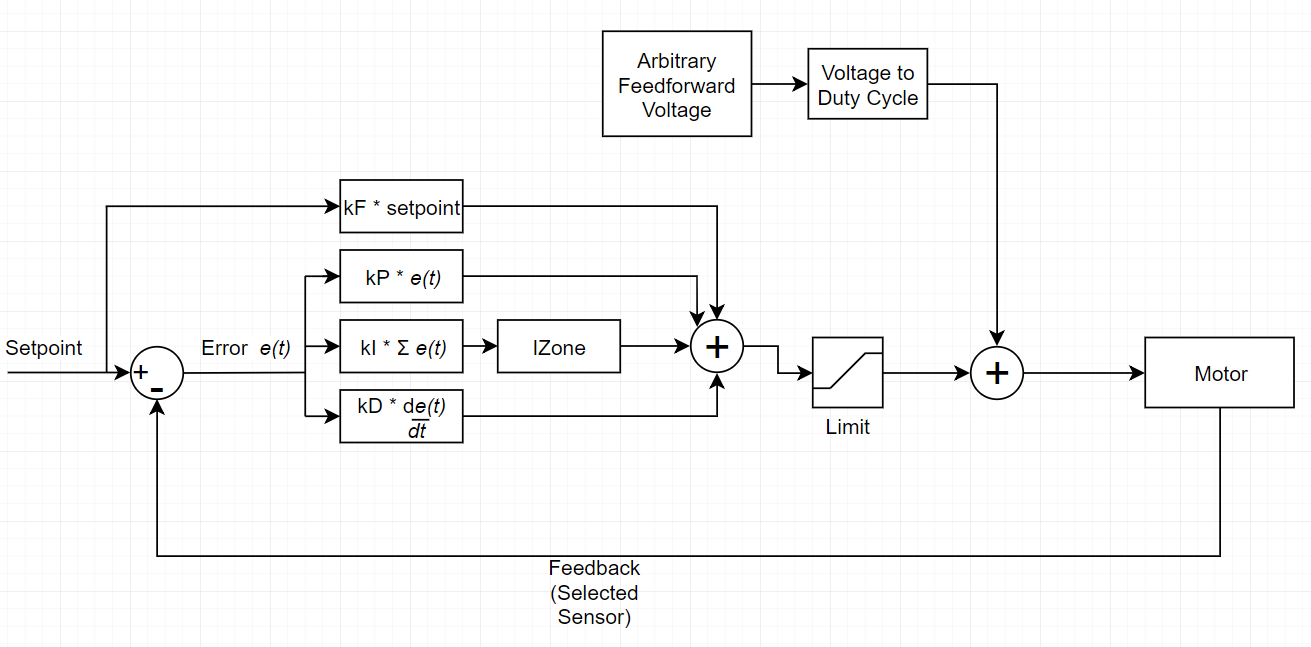Comment on page

# Closed Loop Control

SPARK MAX can operate in several closed-loop control modes, using sensor input to tightly control the motor velocity, position or current. The internal control loop follows a standard PID algorithm with a feed-forward (F) term to compensate for known system offsets.
Additionally, an arbitrary feedforward signal is added to the output of the control loop after all calculations are done. The units for this signal can be selected as either voltage or duty cycle. This feature allows more advanced feedforward calculations to be performed by the controller. More details about the types of feedforward calculations can be found on the WPILib documentation. Using the voltage units for arbitrary feedforward allows the user to send the calculated feedforward voltage from the WPILib API directly to the control loop.
Below is a diagram and the firmware implementation of the internal SPARK MAX PIDF.// Synchronous PID, called at 1kHz.
// The output is always in normalized units (i.e. 1 = full forward, -1 = full reverse).
// The setpoint below is always in native units for the corresponding control method (e.g. rotations, RPM, Amps, Volts),
// after any conversion factors have been applied to the setpoint passed to setReference().
// As a result, the constants have the following units:
// kP, kF: normalized/native
// kI: normalized/(native*ms)
// kD: normalized/(native/ms)
// kMinOutput, kMaxOutput: normalized
// iZone: native
float pid_run(pid_instance_t* pid, float setpoint, float pv,
const pid_constants_t* constants)
{
float error = setpoint - pv;
float p = error * constants->kP;
if(fabsf(error) <= constants->iZone || constants->iZone == 0.0f) {
pid->iState = pid->iState + (error * constants->kI);
} else {
pid->iState = 0;
}
float d = (error - pid->prev_err);
pid->prev_err = error;
d *= constants->kD;
float f = setpoint * constants->kF;
float output = p + pid->iState + d + f;
pid->output = fminf(fmaxf(output,constants->kMinOutput),constants->kMaxOutput);
return output;
}
For more information on utilizing the built-in closed-loop control modes, please take a look at our SPARK MAX Code Examples.